# Arc

Circle arc corresponding to angle is 32° is 28 dm long. What is the length of the entire circle?

Correct result:

l =  315 dm

#### Solution: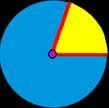We would be pleased if you find an error in the word problem, spelling mistakes, or inaccuracies and send it to us. Thank you!Tips to related online calculators
Check out our ratio calculator.
Do you want to convert length units?

#### You need to know the following knowledge to solve this word math problem:

We encourage you to watch this tutorial video on this math problem:

## Next similar math problems:

• AgesJohn, Teresa, Daniel and Paul have summary 56 years. Their ages are in a ratio of 1:2:5:6. Determine how many years have each of them.
• MineWheel in traction tower has a diameter 4 m. How many meters will perform an elevator cabin if wheel rotates in the same direction 89 times?
• DonutsFind how many donuts each student will receive if you share 126 donuts in a ratio of 1:5:8
• Line segmentThe 4 cm long line segment is enlarged in the ratio of 5/2. How many centimeters will measure the new line segment?
• String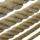Divide the string length 800 cm in ratio 1: 5
• Two gears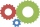The two gears fit together. The larger gear has 32 teeth, the smaller has 20 teeth less. How many times does turn a smaller gear if the bigger gear turns three times?
• Circle r,DCalculate the diameter and radius of the circle if it has length 26.59 cm.
• FlowerbedIn the park there is a large circular flowerbed with a diameter of 12 m. Jakub circulated him ten times and the smaller Vojtoseven times. How many meters each went by and how many meters did Jakub run more than Vojta?
• CircleWhat is the radius of the circle whose perimeter is 6 cm?
• Bridge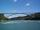The bridge arc has a span 34 m and height 3 m. Calculate the radius of the circle arc of this bridge.
• Wheel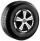What is the wheel diameter if on the 0.38 km track turns 128 times?
• Circumference - a simple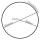What is the ratio of the circumference of any circle and its diameter? Write the result as a real number rounded to 2 decimal places.
• Equator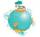Suppose that tourist went on foot over the Globe equator. How many meters more track made ​​his hat on his head as the shoes on your feet? The radius of the earth is 6378 km and height of the tourist is 1.7 m.
• Land areaA land area of Asia and Africa are in a 3: 2 ratio, the European and African are is 1:3. What are the proportions of Asia, Africa, and Europe?
• Wheels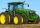Small tractor wheel with a diameter of 60 cm must be rotated 8 times to overcome some pathway. How many times must turn the big tractor wheel with a radius 60 cm to overcome the same distance?
• BaseBase of building is circle with diameter 25 m. Calculate the circumference of a circular trench witch diameter is 41 cm wider than the diameter of the base.
• Arc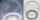The length of the circle is 41 amd arc length of the circle 9. What is the magnitude of the angle of this arc?